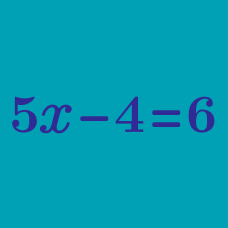Algebra

# Simple Equations

What value of $x$ satisfies the following equation: $x + 5 = 18$?

What is the value of $x$ that satisfies the equation $x+8=40?$

What is the value of $x$ that satisfies the equation $6x = 18$?

If $4x + 11 = 67$, what is the value of $x$?

What is the value of $x$ that satisfies the equation $\dfrac{x}{3} = 9$?

×